Next: Basic Feasible Solutions: An Up: An algebraic characterization of Previous: Example:

#### LP's in ``standard form''

To further exploit the previous characterization of extreme points as the solution to n binding linearly independent constraints, we must define the concept of LP's in ``standard form''. An LP is said to be in ``standard form'', if: (i) all technological constraints are equality constraints, and (ii) all the variables have a nonnegativity sign restriction.

Every LP can be brought into ``standard form'' through the following transformations:

• an inequality constraint: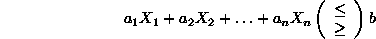can be converted into an equality one, through the introduction of a slack (excess) variable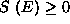: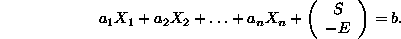• A variable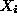with sign restriction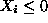can substituted by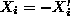with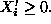• Finally, a urs variablecan be substituted by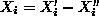with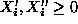.

You can get a clearer idea about how the above transformation works, by having it applied to your own examples. To interpret the obtained results, notice that the final outcome is presented in a "tableau" format.